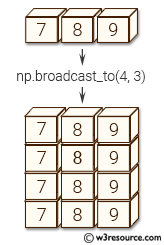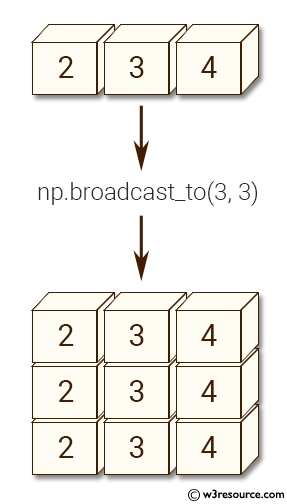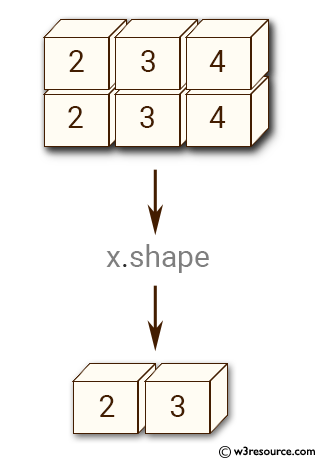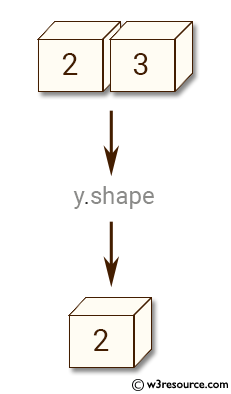# NumPy Array manipulation: broadcast_to() function

## numpy.broadcast_to() function

The broadcast_to() function is used to produce an object that mimics broadcasting.

Syntax:

`numpy.broadcast_to(array, shape, subok=False)`Version: 1.15.0

Parameter:

Name Description Required /
Optional
array The array to broadcast. Required
shape The shape of the desired array. Required
subok If True, then sub-classes will be passed-through, otherwise the returned array will be forced to be a base-class array (default). Optional

Return value:

If True, then sub-classes will be passed-through, otherwise the returned array will be forced to be a base-class array (default).

Raises: ValueError - If the array is not compatible with the new shape according to NumPy’s broadcasting rules.

Example-1: numpy.broadcast_to()

``````>>> import numpy as np
>>> a = np.array([2,3,4])
>>> np.broadcast_to(a, (3, 3))
array([[2, 3, 4],
[2, 3, 4],
[2, 3, 4]])
``````

Pictorial Presentation:Example-2: numpy.broadcast_to()

``````>>> import numpy as np
>>> from numpy import array
>>> x = array([[2, 3, 4], [2, 3, 4]])
>>> print(x.shape)
(2, 3)
``````

Pictorial Presentation:Example-3: numpy.broadcast_to()

``````>>> import numpy as np
>>> y = array([2, 3])
>>> print(y.shape)
(2,)
``````

Pictorial Presentation:Example-4: numpy.broadcast_to()

``````>>> import numpy as np
>>> x = np.ma.array([2, 3, 4], mask=[False, True, False])
>>> y = np.broadcast_to(x, (3, 3), subok=True)
>>> y.mask
False
>>> y.mask = np.broadcast_to(x.mask, y.shape)
>>> y
masked_array(data =
[[2 -- 4]
[2 -- 4]
[2 -- 4]],
mask =
[[False  True False]
[False  True False]
[False  True False]],
fill_value = 999999)
``````

Python - NumPy Code Editor:

Previous: broadcast
Next: broadcast_arrays()

﻿